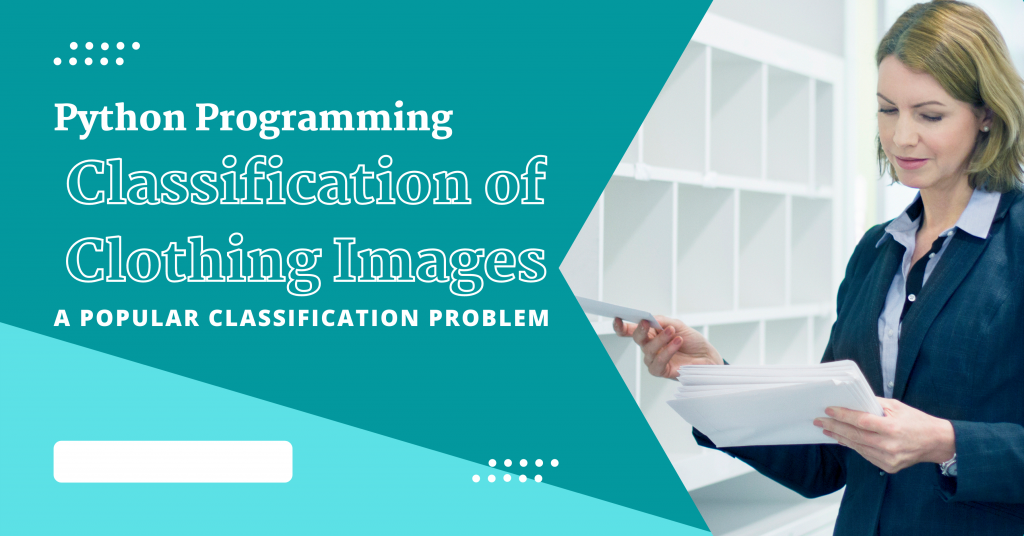# Classifying Clothing Images in Python – A complete guideHello, folks! In this tutorial, we’ll have a look at how the Classification of various clothing images takes place with the help of TensorFlow using the Python programming language.

The social media platforms Instagram, YouTube, and Twitter have taken over our daily lives. Models and celebrities, in particular, need to know how to categorize clothing into several categories if they want to look their best.

Also read: Crypto Price Prediction with Python

The classification of fashion items in a photograph includes the identification of individual garments. The same has applications in social networking, e-commerce, and criminal law as well.

## Step 1: Importing modules

The first step in every project is to import all the required modules. We’ll be working with Tensorflow along with numpy and matplotlib.

```import tensorflow as tf
import numpy as np
import matplotlib.pyplot as plt
```

The dataset that we are going to load into our program can be seen here.

This dataset includes `60,000` photos in grayscale, each measuring `28x28 pixels`, from ten different fashion categories, plus a dummy set of `10,000 `images.

MNIST can be replaced using this dataset. The code line below achieves the loading of data.

```fashion_data=tf.keras.datasets.fashion_mnist
```

## Step 3: Training and Testing Data Split

A major part of any Machine Learning model includes dividing the data into two parts based on the 80-20 rule.

The 80-20 rule states that 80% of the data is sent to training data and 20% to testing data. The code below splits the data into training and testing.

```(inp_train,out_train),(inp_test,out_test)=fashion_data.load_data()
inp_train = inp_train/255.0
inp_test = inp_test/255.0
print("Shape of Input Training Data: ", inp_train.shape)
print("Shape of Output Training Data: ", out_train.shape)
print("Shape of Input Testing Data: ", inp_test.shape)
print("Shape of Output Testing Data: ", out_test.shape)
```

The code alsonormalizes the dataset loaded.

```Shape of Input Training Data:  (60000, 28, 28)
Shape of Output Training Data:  (60000,)
Shape of Input Testing Data:  (10000, 28, 28)
Shape of Output Testing Data:  (10000,)
```

## Step 4: Data Visualization

The code to visualize the initial data is as follows:

```plt.figure(figsize=(10,10))
for i in range(100):
plt.subplot(10,10,i+1)
plt.imshow(inp_train[i])
plt.xticks([])
plt.yticks([])
plt.xlabel(out_train[i])
plt.tight_layout()
plt.show()
```

## Step 5: Changing the labels to actual names

We have seen the visualization, but we also want the labels to have well-defined names. The code mentioned below will achieve the purpose.

```Labels=['T-shirt/top', 'Trouser', 'Pullover', 'Dress', 'Coat','Sandal', 'Shirt', 'Sneaker', 'Bag', 'Ankle boot']
plt.figure(figsize=(10,10))
for i in range(100):
plt.subplot(10,10,i+1)
plt.xticks([])
plt.yticks([])
plt.imshow(inp_train[i], cmap=plt.cm.binary)
plt.xlabel(Labels[out_train[i]])
plt.tight_layout()
plt.show()
```

You can see now that the visualization is now more understandable.

## Step 6: Building, Compiling, and Training the model

The code for the building, compiling, and training of the TensorFlow and Keras model is displayed below:

```my_model = tf.keras.Sequential([
tf.keras.layers.Flatten(input_shape=(28, 28)),
tf.keras.layers.Dense(128, activation='relu'),
tf.keras.layers.Dense(10)
])
loss=tf.keras.losses.SparseCategoricalCrossentropy(from_logits=True),
metrics=['accuracy'])
my_model.fit(inp_train, out_train, epochs=20)
```

## Step 7: Checking the final loss and accuracy

Now that our model is trained successfully, it now turns to compute the loss and find the final accuracy of the model created and trained.

```loss, accuracy = my_model.evaluate(inp_test,out_test,verbose=2)
print('\nAccuracy:',accuracy*100)
```

The final accuracy we get after the whole processing of our model is 88.8% which is pretty good.

## Step8: Make Predictions

We have come to the final step of the program that is making predictions using the model we just created and trained.

```prob=tf.keras.Sequential([my_model,tf.keras.layers.Softmax()])
pred=prob.predict(inp_test)
```

## Step 9: Visualizing the final predictions

It is important for any classification model, that we make the final visualization. To make this simpler we will be visualizing the first 20 images of the dataset.

```plt.figure(figsize=(20,20))
for i in range(20):
true_label,image = out_test[i],inp_test[i]
pred_label = np.argmax(pred[i])
plt.subplot(10,10,i+1)
plt.xticks([])
plt.yticks([])
plt.imshow(image, cmap=plt.cm.binary)
if pred_label == true_label:
color = 'green'
label="Correct Prediction!"
else:
color = 'red'
label="Wrong Prediction!"
plt.tight_layout()
plt.title(label,color=color)
plt.xlabel(" {} -> {} ".format(Labels[true_label],Labels[pred_label]))
```